Output Data Sets

The VARIOGRAM procedure produces four data sets: the OUTACWEIGHTS=SAS-data-set, the OUTDIST=SAS-data-set, the OUTPAIR=SAS-data-set, and the OUTVAR=SAS-data-set. These data sets are described in the following sections.

### OUTACWEIGHTS=SAS-data-set

The OUTACWEIGHTS= data set contains one observation for each pair of pointsin the original data set, where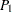is different from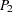, with information about the data distance and autocorrelation weight of each point pair.

The OUTACWEIGHTS= data set can be very large, even for a moderately sized DATA= data set. For example, if the DATA= data set has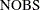=500, then the OUTACWEIGHTS= data set has=124,750 observations.

When you perform autocorrelation computations, the OUTACWEIGHTS= data set is a practical way to save the autocorrelation weights for further use.

The OUTACWEIGHTS= data set contains the following variables:

• ACWGHT12, the autocorrelation weight for the pair• ACWGHT21, the autocorrelation weight for the pair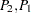• DISTANCE, the distance between the data in the pair

• ID1, the ID variable value or observation number for the first point in the pair

• ID2, the ID variable value or observation number for the second point in the pair

• V1, the variable value for the first point in the pair

• V2, the variable value for the second point in the pair

• VARNAME, the variable name for the current VAR variable

• X1, thecoordinate of the first point in the pair

• X2, thecoordinate of the second point in the pair

• Y1, thecoordinate of the first point in the pair

• Y2, thecoordinate of the second point in the pair

When the autocorrelation weights are symmetric, the pairhas the same weight as the pair. For this reason, in the case of symmetric weights the OUTACWEIGHTS= data set contains only the autocorrelation weights ACWGHT12.

If no ID statement is specified, then the corresponding observation number is assigned to each one of the variables ID1 and ID2, instead.

### OUTDIST=SAS-data-set

The OUTDIST= data set contains counts for a modified histogram that shows the distribution of pairwise distances. This data set provides you with information related to the choice of values for the LAGDISTANCE= option in the COMPUTE statement.

To request an OUTDIST= data set, specify the OUTDIST= data set in the PROC VARIOGRAM statement and the NOVARIOGRAM option in the COMPUTE statement. The NOVARIOGRAM option prevents any semivariogram or covariance computation from being performed.

The following variables are written to the OUTDIST= data set:

• COUNT, the number of pairs that fall into this lag class

• LAG, the lag class value

• LB, the lower bound of the lag class interval

• UB, the upper bound of the lag class interval

• PER, the percent of all pairs that fall in this lag class

• VARNAME, the name of the current VAR variable

### OUTMORAN=SAS-data-set

The OUTMORAN= data set contains the standardized value (or response) of each observation and the weighted average of its N neighbors, based on a neighborhood within a LAGDISTANCE= distance from the observation. To request this data set, specify the OUTMORAN= data set in the PROC VARIOGRAM statement, in addition to the AUTOCORRELATION and LAGDISTANCE= options in the COMPUTE statement.

The following variables are written to the OUTMORAN= data set:

• DISTANCE, the value of the neighborhood radius, which is specified with the LAGDISTANCE= option

• ID, the ID variable value or observation number for the current observation

• N, the number of neighbors within the specified DISTANCE from the current observation

• RESPONSE, the standardized value of the current observation

• STDWAVG, the standardized weighted average of the neighbors for the current observation

• V, the variable value of the current observation

• VARNAME, the variable name for the current VAR variable

• X, thecoordinate of the current observation

• Y, thecoordinate of the current observation

• WAVG, the weighted average of the neighbors for the current observation

For zero neighbors in the neighborhood of a nonmissing observation, the corresponding value of the variable N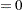and the variables STDWAVG and WAVG are assigned missing values. Observations with missing values are included in the OUTMORAN= data set if they have neighbors and only if nonmissing observations with neighbors also exist in the same data set.

### OUTPAIR=SAS-data-set

When you specify the NOVARIOGRAM option in the COMPUTE statement, the OUTPAIR= data set contains one observation for each distinct pair of pointsin the original data set. Otherwise, the OUTPAIR= data set might have fewer observations, depending on the values you specify in the LAGDISTANCE= and MAXLAGS= options and whether you specify the OUTPDISTANCE= option in the COMPUTE statement.

If the NOVARIOGRAM option is not specified in the COMPUTE statement, then the OUTPAIR= data set contains one observation for each distinct pair of points that are up to a distance within MAXLAGS= away from each other. If you also specify the OUTPDISTANCE=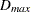option in the COMPUTE statement, then all pairsin the original data set that satisfy the relationare written to the OUTPAIR= data set.

Given the aforementioned specifications, note that the OUTPAIR= data set can be very large even for a moderately sized DATA= data set. For example, if the DATA= data set has=500, then the OUTPAIR= data could have up to=124,750 observations if no OUTPDISTANCE= restriction is given in the COMPUTE statement.

The OUTPAIR= data set contains information about the distance and orientation of each point pair, and you can use it for specialized continuity measure calculations.

The OUTPAIR= data set contains the following variables:

• AC, the angle class value

• COS, the cosine of the angle between pairs

• DC, the distance (lag) class

• DISTANCE, the distance between the data in pairs

• ID1, the ID variable value or observation number for the first point in the pair

• ID2, the ID variable value or observation number for the second point in the pair

• V1, the variable value for the first point in the pair

• V2, the variable value for the second point in the pair

• VARNAME, the variable name for the current VAR variable

• X1, thecoordinate of the first point in the pair

• X2, thecoordinate of the second point in the pair

• Y1, thecoordinate of the first point in the pair

• Y2, thecoordinate of the second point in the pair

If no ID statement is specified, then the corresponding observation number is assigned to each one of the variables ID1 and ID2, instead.

### OUTVAR=SAS-data-set

The OUTVAR= data set contains the standard and robust versions of the sample semivariance, the covariance, and other information in each lag class.

The OUTVAR= data set contains the following variables:

• ANGLE, the angle class value (clockwise from N to S)

• ATOL, the angle tolerance for the lag or angle class

• AVERAGE, the average variable value for the lag or angle class

• BANDW, the bandwidth for the lag or angle class

• COUNT, the number of pairs in the lag or angle class

• COVAR, the covariance value for the lag or angle class

• DISTANCE, the average lag distance for the lag or angle class

• LAG, the lag class value (in LAGDISTANCE= units)

• RVARIO, the sample robust semivariance value for the lag or angle class

• STDERR, the approximate standard error of the sample semivariance estimate

• VARIOG, the sample semivariance value for the lag or angle class

• VARNAME, the name of the current VAR variable

The robust semivariance estimate, RVARIO, is not included in the data set if you omit the option ROBUST in the COMPUTE statement.

The bandwidth variable, BANDW, is not included in the data set if no bandwidth specification is given in the COMPUTE statement or in a DIRECTIONS statement.

The OUTVAR= data set contains a line where the LAG variable is. The AVERAGE variable in this line displays the sample mean valueof the SRF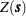, and the COVAR variable shows the sample variance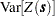.